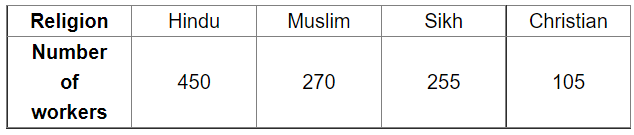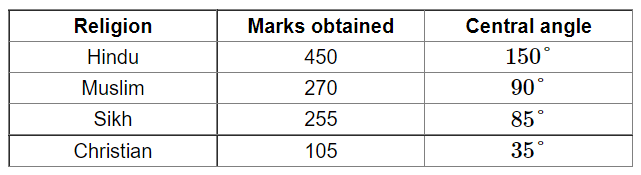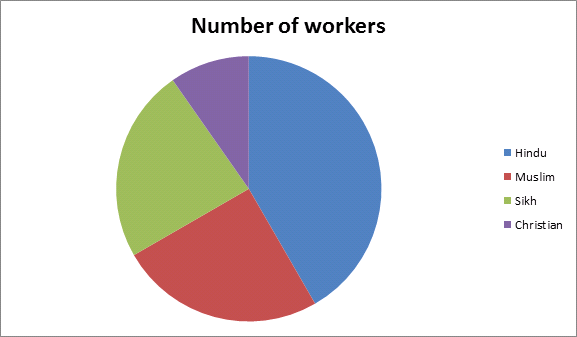# The data on religion wise division of 1080 workers of a factory are given below:

Question:

The data on religion wise division of 1080 workers of a factory are given below:Draw a pie chart to represent the above data.

Solution:

Total number of workers $=1080$

Central angle of each religion $=\left(\frac{\text { number of workers in each religion }}{\text { total number of workers }} \times 360\right)^{\circ}$

Calculation of central anglesConstruction of pie chart

Steps of construction:

1. Draw a circle of any convenient radius.

2. Draw a horizontal radius of this circle.

3. Draw sectors whose central angles are $150^{\circ}, 90^{\circ}, 85^{\circ}$ and $35^{\circ}$.

4. Shade the sectors so obtained differently and label each one of them.

Thus, we obtain the required pie chart as shown in the figure below.